# Java Multithreading Tutorial

• Difficulty Level : Medium
• Last Updated : 03 Aug, 2022

Threads are the backbone of multithreading. We are living in a real-world which in itself is caught on the web surrounded by lots of applications. With the advancement in technologies we cannot achieve the speed required to run them simultaneously unless we introduce the concept of multi tasking efficiently. It is achieved by the concept of thread.Real-life Example

Suppose you are using two tasks at a time on the computer, be it using Microsoft Word and listening to music. These two tasks are called processes. So you start typing in Word and at the same time start music app, this is called multitasking. Now you committed a mistake in a Word and spell check shows exception, this means Word is a process that is broken down into sub-processes. Now if a machine is dual-core then one process or task is been handled by one core and music is been handled by another core.

In the above example, we come across both multiprocessing and multithreading. These are somehow indirectly used to achieve multitasking. In this way the mechanism of dividing the tasks is called multithreading in which every process or task is called by a thread where a thread is responsible for when to execute, when to stop and how long to be in a waiting state. Hence, a thread is the smallest unit of processing whereas multitasking is a process of executing multiple tasks at a time.

Multitasking is being achieved in two ways:

1. Multiprocessing: Process-based multitasking is a heavyweight process and occupies different address spaces in memory. Hence, while switching from one process to another, it will require some time be it very small, causing a lag because of switching. This happens as registers will be loaded in memory maps and the list will be updated.
2. Multithreading: Thread-based multitasking is a lightweight process and occupies the same address space. Hence, while switching cost of communication will be very less.

Below is the Lifecycle of a Thread been illustrated

1. New: When a thread is just created.
2. Runnable: When a start() method is called over thread processed by the thread scheduler.
• Case A: Can be a running thread
• Case B: Can not be a running thread
3. Running: When it hits case 1 means the scheduler has selected it to be run the thread from runnable state to run state.
4. Blocked: When it hits case 2 meaning the scheduler has selected not to allow a thread to change state from runnable to run.
5. Terminated: When the run() method exists or stop() method is called over a thread.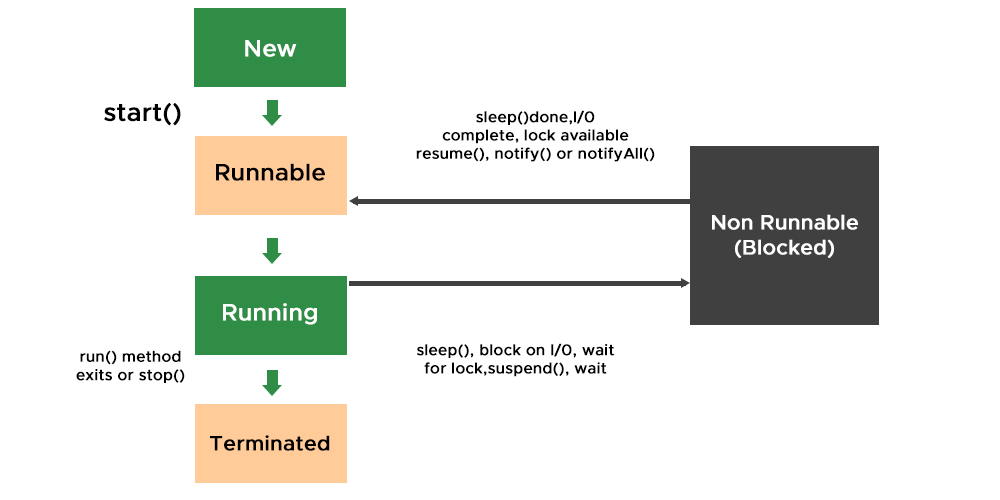If we do incorporate threads in operating systems one can perceive that the process scheduling algorithms in operating systems are strongly deep-down working on the same concept incorporating thread in Gantt charts. A few of the most popular are listed below which wraps up all of them and are used practically in software development.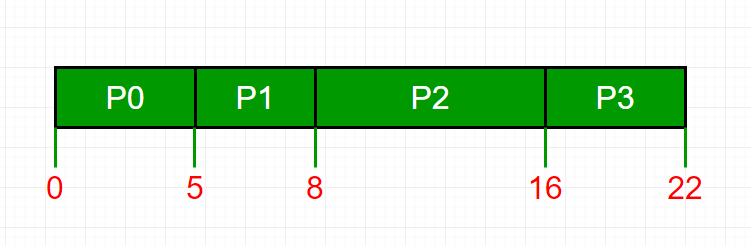• First In First Out
• Last In First Out
• Round Robin Scheduling

Now Imagine the concept of Deadlock in operating systems with threads – how the switching is getting computed over internally if one only has an overview of them.

So far we have understood multithreading and thread conceptually, so we can conclude advantages of multithreading before moving to any other concept or getting to programs in multithreading.

• The user is not blocked as threads are independent even if there is an issue with one thread then only the corresponding process will be stopped rest all the operations will be computed successfully.
• Saves time as too many operations are carried over at the same time causing work to get finished as if threads are not used the only one process will be handled by the processor.
• Threads are independent though sharing the same address space.

So we have touched all main concepts of multithreading but the question striving in the head is left. why do we need it, where to use it and how? Now, we will discuss all three scenarios why multithreading is needed and where it is implemented via the help of programs in which we will be further learning more about threads and their methods. We need multithreading in four scenarios as listed.

• Mobile applications
• Web applications
• Game Development

Note: By default we only have one main thread which is responsible for main thread exception as you have encountered even without having any prior knowledge of multithreading

### Method 1: Using Thread Class

Java provides Thread class to achieve programming invoking threads thereby some major methods of thread class are shown below in the tabular format with which we deal frequently along the action performed by them.

Pre-requisites: Basic syntax and methods to deal with threads

Now let us come up with how to set the name of the thread. By default, threads are named thread-0, thread-1, and so on. But there is also a method that is often used  as setName() method. Also corresponding to it there is a method getName() which returns the name of the thread be it default or settled already by using setName() method. The syntax is as follows:

Syntax:

(a) Returning the name of the thread

`public String getName() ;`

(b) Changing the name of the thread

` public void setName(String name);`

Taking a step further, let us dive into the implementation part to understand more concepts about multithreading. So, there are basically two ways of implementing multithreading:

Illustration: Consider if one has to multiply all elements by 2 and there are 500 elements in an array.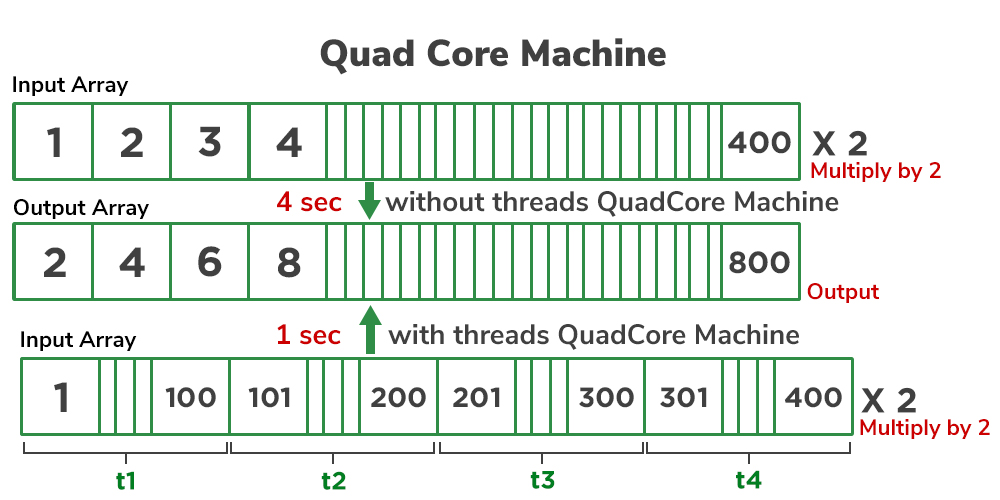Examples

## Java

 `// Case 1``// Java Program to illustrate Creation and execution of``// thread via start() and run() method in Single inheritance` `// Class 1``// Helper thread Class extending main Thread Class``class` `MyThread1 ``extends` `Thread {` `    ``// Method inside MyThread2``    ``// run() method which is called as``    ``// soon as thread is started``    ``public` `void` `run()``    ``{` `        ``// Print statement when the thread is called``        ``System.out.println(``"Thread1 is running"``);``    ``}``}` `// Class 2``// Main thread Class extending main Thread Class``class` `MyThread2 ``extends` `Thread {` `    ``// Method inside MyThread2``    ``// run() method which is called``    ``// as soon as thread is started``    ``public` `void` `run()``    ``{` `        ``// run() method which is called as soon as thread is``        ``// started` `        ``// Print statement when the thread is called``        ``System.out.println(``"Thread2 is running"``);``    ``}``}` `// Class 3``// Main Class``class` `GFG {` `    ``// Main method``    ``public` `static` `void` `main(String[] args)``    ``{` `        ``// Creating a thread object of our thread class``        ``MyThread1 obj1 = ``new` `MyThread1();``        ``MyThread2 obj2 = ``new` `MyThread2();` `        ``// Getting the threads to the run state` `        ``// This thread will transcend from runnable to run``        ``// as start() method will look for run() and execute``        ``// it``        ``obj1.start();` `        ``// This thread will also transcend from runnable to``        ``// run as start() method will look for run() and``        ``// execute it``        ``obj2.start();``    ``}``}`

## Java

 `// Case 2``// Java Program to illustrate Difference between Runnable``// & Non-runnable Threads And Single Inheritance` `// Class 1``// Helper thread Class extending main Thread Class``class` `MyThread1 ``extends` `Thread {` `    ``// Method inside MyThread2``    ``// run() method which is called as soon as thread is``    ``// started``    ``public` `void` `run() {` `        ``// Print statement when the thread is called``        ``System.out.println(``"Thread 1 is running"``);``    ``}``}` `// Class 2``// Main thread Class extending main Thread Class``class` `MyThread2 ``extends` `Thread {` `    ``// Method``    ``public` `void` `show() {` `        ``// Print statement when thread is called``        ``System.out.println(``"Thread 2"``);``    ``}``}` `// Class 3``// Main Class``class` `GFG {` `    ``// Main method``    ``public` `static` `void` `main(String[] args) {` `        ``// Creating a thread object of our thread class``        ``MyThread1 obj1 = ``new` `MyThread1();``        ``MyThread2 obj2 = ``new` `MyThread2();` `        ``// Getting the threads to the run state` `        ``// This thread will transcend from runnable to run``        ``// as start() method will look for run() and execute``        ``// it``        ``obj1.start();` `        ``// This thread will now look for run() method which is absent``        ``// Thread is simply created not runnable``        ``obj2.start();``    ``}``}`

## Java

 `// Java Program to illustrate difference between``// start() method thread vs show() method` `// Class 1``// Helper thread Class extending main Thread Class``class` `MyThread1 ``extends` `Thread {` `    ``// Method inside MyThread2``    ``// run() method which is called as soon as thread is``    ``// started``    ``public` `void` `run() {` `        ``// Print statement when the thread is called``        ``System.out.println(``"Thread 1 is running"``);``    ``}``}` `// Class 2``// Main thread Class extending main Thread Class``class` `MyThread2 ``extends` `Thread {` `    ``// Method``    ``public` `void` `show() {` `        ``// Print statement when thread is called``        ``System.out.println(``"Thread 2"``);``    ``}``}` `// Class 3``// Main Class``class` `GFG {` `    ``// Main method``    ``public` `static` `void` `main(String[] args) {` `        ``// Creating a thread object of our thread class``        ``MyThread1 obj1 = ``new` `MyThread1();``        ``MyThread2 obj2 = ``new` `MyThread2();` `        ``// Getting the threads to the run state` `        ``// This thread will transcend from runnable to run``        ``// as start() method will look for run() and execute``        ``// it``        ``obj1.start();` `        ``// This thread is simply a function call as``        ``// no start() method is executed so here only``        ``// thread is created only followed by call``        ``obj2.show();``    ``}``}`

### Output:

Case 1:

```Thread1 is running

Here we have created our two thread classes for each thread. In the main method, we are simply creating objects of these thread classes where objects are now threads. So in main, we call thread using start() method over both the threads. Now start() method starts the thread and lookup for their run() method to run. Here both of our thread classes were having run()  methods, so both threads are put to run state from runnable by the scheduler, and output on the console is justified.

Case 2:

`Thread 1 is running`

Here we have created our two thread classes for each thread. In the main method, we are simply creating objects of these thread classes where objects are now threads. So in main, we call thread using start() method over both the threads. Now start() method starts the thread and lookup their run() method to run. Here only class 1 is having the run() method to make the thread transcend from runnable to run state to execute whereas thread 2 is only created but not put to run state by the scheduler as its corresponding run() method was missing. Hence, only thread 1 is called rest thread 2 is created only and is in the runnable state later blocked by scheduler because the corresponding run() method was missing.

Case 3:

```Thread 2
Thread 1 is running```

### Method 2: Using Runnable Interface

Another way to achieve multithreading in java is via the Runnable interface. Here as we have seen in the above example in way 1 where Thread class is extended. Here Runnable interface being a functional interface has its own run() method. Here classes are implemented to the Runnable interface. Later on, in the main() method, Runnable reference is created for the classes that are implemented in order to make bondage with Thread class to run our own corresponding run() methods. Further, while creating an object of Thread class we will pass these references in Thread class as its constructor allows only one runnable object, which is passed as a parameter while creating Thread class object in a main() method. Now lastly just like what we did in Thread class, start() method is invoked over the runnable object who are now already linked with Thread class objects, so the execution begins for our run() methods in case of Runnable interface. It is shown in the program below as follows:

Example:

## Java

 `// Java Program to illustrate Runnable Interface in threads``// as multiple inheritance is not allowed` `// Importing basic packages``import` `java.io.*;``import` `java.util.*;` `// Class 1``// Helper class implementing Runnable interface``class` `MyThread1 ``implements` `Runnable {` `    ``// run() method inside this class``    ``public` `void` `run()``    ``{``        ``// Iterating to get more execution of threads``        ``for` `(``int` `i = ``0``; i < ``5``; i++) {` `            ``// Print statement whenever run() method``            ``// of this class is called``            ``System.out.println(``"Thread1"``);` `            ``// Getting sleep method in try block to``            ``// check for any exceptions``            ``try` `{``                ``// Making the thread pause for a certain``                ``// time using sleep() method``                ``Thread.sleep(``1000``);``            ``}` `            ``// Catch block to handle the exceptions``            ``catch` `(Exception e) {``            ``}``        ``}``    ``}``}` `// Class 2``// Helper class implementing Runnable interface``class` `MyThread2 ``implements` `Runnable {` `    ``// run() method inside this class``    ``public` `void` `run()``    ``{``        ``for` `(``int` `i = ``0``; i < ``5``; i++) {` `            ``// Print statement whenever run() method``            ``// of this class is called``            ``System.out.println(``"Thread2"``);` `            ``// Getting sleep method in try block to``            ``// check for any exceptions``            ``try` `{` `                ``// Making the thread pause for a certain``                ``// time``                ``// using sleep() method``                ``Thread.sleep(``1000``);``            ``}` `            ``// Catch block to handle the exceptions``            ``catch` `(Exception e) {``            ``}``        ``}``    ``}``}` `// Class 3``// Main class``public` `class` `GFG {` `    ``// Main driver method``    ``public` `static` `void` `main(String[] args)``    ``{` `        ``// Creating reference of Runnable to``        ``// our classes above in main() method``        ``Runnable obj1 = ``new` `MyThread1();``        ``Runnable obj2 = ``new` `MyThread2();` `        ``// Creating reference of thread class``        ``// by passing object of Runnable in constructor of``        ``// Thread class``        ``Thread t1 = ``new` `Thread(obj1);``        ``Thread t2 = ``new` `Thread(obj2);` `        ``// Starting the execution of our own run() method``        ``// in the classes above``        ``t1.start();``        ``t2.start();``    ``}``}`

Output

```Thread2

Points to remember: Whenever you wanted to create threads, there are only two ways:

1. Extending the class
2. Implementing the interface which is runnable

Make sure to create an object of threads in which you have to pass the object of runnable

### Special Methods of Threads

Now let us discuss various methods that are there for threads. Here we will be discussing major methods in order to have a practical understanding of threads and multithreading which are sequential as follows:

1. start() Method
2. suspend() Method
3. stop() Method
4. wait() Method
5. notify() Method
6. notifyAll() Method
7. sleep() Method
• Output Without sleep() Method
• Output with sleep() method in Serial Execution Processes (Blocking methods approach)
• Output with sleep() method in Parallel Execution Processes (Unblocking methods approach)
8. join() Method

Note: For naive users in multithreading where threads are backbone go through Program 4 to get very basics of threads, how to start, make it hold, or terminate then only toggle to program 1 and rest as follows.

Implementation:

## Java

 `// Example 1``// Java Program to illustrate Output Without sleep() Method` `// Class 1``// Helper Class 1``class` `Shot ``extends` `Thread {` `    ``// Method 1``    ``public` `void` `show() {` `        ``// Iterating to print more number of times``        ``for` `(``int` `i = ``0``; i < ``5``; i++) {` `            ``// Print statement whenever method``            ``// of this class is called``            ``System.out.println(``"Shot"``);` `        ``}``    ``}``}` `// Class 2``// Helper Class 2``class` `Miss ``extends` `Thread {` `    ``// Method 2``    ``public` `void` `show() {` `        ``// Iterating to print more number of times``        ``for` `(``int` `i = ``0``; i < ``5``; i++) {` `            ``// Print statement whenever method``            ``// of this class is called``            ``System.out.println(``"Miss"``);` `        ``}``    ``}` `}` `// Class 3``// Main class``public` `class` `GFG {` `    ``// Method 3``    ``// Main method``    ``public` `static` `void` `main(String[] args) {` `        ``// Creating objects in the main() method``        ``// of class 1 and class 2``        ``Shot obj1 = ``new` `Shot();``        ``Miss obj2 = ``new` `Miss();` `        ``// Calling methods of the class 1 and class 2``        ``obj1.show();``        ``obj2.show();` `    ``}``}`

## Java

 `// Example 2``// Java Program to illustrate Output Using sleep() Method``// in Serial Execution` `// Class 1``// Helper Class 1``class` `Shot ``extends` `Thread {` `    ``// Method 1``    ``// public void show() {``    ``public` `void` `show()``    ``{` `        ``// Iterating to print more number of times``        ``for` `(``int` `i = ``0``; i < ``5``; i++) {` `            ``// Print statement``            ``System.out.println(``"Shot"``);` `            ``// Making thread to sleep using sleep() method` `            ``// Try-catch block for exceptions``            ``try` `{``                ``Thread.sleep(``1000``);``            ``}``            ``catch` `(Exception e) {``            ``}``        ``}``    ``}``}` `// Class 2``// Helper Class 2 Hello``class` `Miss ``extends` `Thread {` `    ``// Method 2``    ``// public void show() {``    ``public` `void` `show()``    ``{` `        ``// Iterating to print more number of times``        ``for` `(``int` `i = ``0``; i < ``5``; i++) {` `            ``// Print statement``            ``System.out.println(``"Miss"``);` `            ``// Making thread to sleep using sleep() method` `            ``// Try-catch block for exceptions``            ``try` `{``                ``Thread.sleep(``1000``);``            ``}``            ``catch` `(Exception e) {``            ``}``        ``}``    ``}``}` `// Class 3``// Main class``public` `class` `GFG {` `    ``// Method 3``    ``// Main method``    ``public` `static` `void` `main(String[] args)``    ``{` `        ``// Creating objects in the main() method``        ``Shot obj1 = ``new` `Shot();``        ``Miss obj2 = ``new` `Miss();` `        ``// Starting the thread objects``        ``obj1.start();``        ``obj2.start();` `        ``// Calling methods of class 1 and class 2``        ``obj1.show();``        ``obj2.show();``    ``}``}`

## Java

 `// Example 3``// Java Program to illustrate Output Using sleep() Method``// in Parallel Execution` `// Class 1``// Helper Class 1``class` `Shot ``extends` `Thread {` `    ``// Method 1``    ``// public void show() {``    ``public` `void` `run()``    ``{` `        ``// Iterating to print more number of times``        ``for` `(``int` `i = ``0``; i < ``5``; i++) {` `            ``// Print statement``            ``System.out.println(``"Shot"``);` `            ``// Making thread to sleep using sleep() method` `            ``// Try catch block for exceptions``            ``try` `{``                ``Thread.sleep(``1000``);``            ``}``            ``catch` `(Exception e) {``            ``}``        ``}``    ``}``}` `// Class 2``// Helper Class 2 Hello``class` `Miss ``extends` `Thread {` `    ``// Method 2``    ``// public void show() {``    ``public` `void` `run()``    ``{` `        ``// Iterating to print more number of times``        ``for` `(``int` `i = ``0``; i < ``5``; i++) {` `            ``// Print statement``            ``System.out.println(``"Miss"``);` `            ``// Making thread to sleep using sleep() method` `            ``// Try catch block for exceptions``            ``try` `{``                ``Thread.sleep(``1000``);``            ``}``            ``catch` `(Exception e) {``            ``}``        ``}``    ``}``}` `// Class 3``// Main class``public` `class` `GFG {` `    ``// Method 3``    ``// Main method``    ``public` `static` `void` `main(String[] args)``    ``{` `        ``// Creating objects in the main() method``        ``Shot obj1 = ``new` `Shot();``        ``Miss obj2 = ``new` `Miss();` `        ``// Starting the thread objects``        ``// using start() method` `        ``// start() method calls the run() method``        ``// automatically``        ``obj1.start();``        ``obj2.start();``    ``}``}`

Output:

Case 1:

```Shot
Shot
Shot
Shot
Shot
Miss
Miss
Miss
Miss
Miss```

Case 2: Video output

```Shot
Shot
Shot
Shot
Shot
Miss
Miss
Miss
Miss
Miss```

Case 3: Video output

```Shot
Miss
Shot
Miss
Shot
Miss
Shot
Miss
Shot
Miss```

Note: There is no priority been set for threads for which as per the order of execution of threads outputs will vary so do remember this drawback of multithreading of different outputs leading to data inconsistency issues which we will be discussing in-depth in the later part under synchronization in threads.

### Priorities in Threads

Priorities in threads is a concept where each thread is having a priority which is represented by numbers ranging from 1 to 10.

• The default priority is set to 5 as excepted.
• Minimum priority is set to 0.
• Maximum priority is set to 10.

Here 3 constants are defined in it namely as follows:

1. public static int NORM_PRIORITY
2. public static int MIN_PRIORITY
3. public static int MAX_PRIORITY

Let us discuss it with an example to get how internally the work is getting executed. Here we will be using the knowledge gathered above as follows:

• We will use currentThread() method to get the name of the current thread. User can also use setName() method if he/she wants to make names of thread as per choice for understanding purposes.
• getName() method will be used to get the name of the thread.

## Java

 `// Java Program to illustrate Priority Threads``// Case 1: No priority is assigned (Default priority)` `// Importing input output thread class``import` `java.io.*;``// Importing Thread class from java.util package``import` `java.util.*;` `// Class 1``// Helper Class (Our thread  class)``class` `MyThread ``extends` `Thread {` `    ``public` `void` `run()``    ``{` `        ``// Printing the current running thread via getName()``        ``// method using currentThread() method``        ``System.out.println(``"Running Thread : "``                           ``+ currentThread().getName());` `        ``// Print and display the priority of current thread``        ``// via currentThread() using getPriority() method``        ``System.out.println(``"Running Thread Priority : "``                           ``+ currentThread().getPriority());``    ``}``}` `// Class 2``// Main Class``class` `GFG {` `    ``// Main driver method``    ``public` `static` `void` `main(String[] args)``    ``{` `        ``// Creating objects of MyThread(above class)``        ``// in the main() method``        ``MyThread t1 = ``new` `MyThread();``        ``MyThread t2 = ``new` `MyThread();` `        ``// Case 1: Default Priority no setting``        ``t1.start();``        ``t2.start();``    ``}``}`

## Java

 `// Java Program to illustrate Priority Threads``// Case 2: NORM_PRIORITY` `// Importing input output thread class``import` `java.io.*;``// Importing Thread class from java.util package``import` `java.util.*;` `// Class 1``// Helper Class (Our thread  class)``class` `MyThread ``extends` `Thread {` `    ``// run() method to transit thread from``    ``// runnable to run state``    ``public` `void` `run()``    ``{` `        ``// Printing the current running thread via getName()``        ``// method using currentThread() method``        ``System.out.println(``"Running Thread : "``                           ``+ currentThread().getName());` `        ``// Print and display the priority of current thread``        ``// via currentThread() using getPriority() method``        ``System.out.println(``"Running Thread Priority : "``                           ``+ currentThread().getPriority());``    ``}``}` `// Class 2``// Main Class``class` `GFG {` `    ``// Main driver method``    ``public` `static` `void` `main(String[] args)``    ``{` `        ``// Creating objects of MyThread(above class)``        ``// in the main() method``        ``MyThread t1 = ``new` `MyThread();``        ``MyThread t2 = ``new` `MyThread();` `        ``// Setting priority to thread via NORM_PRIORITY``        ``// which set priority to 5 as default thread``        ``t1.setPriority(Thread.NORM_PRIORITY);``        ``t2.setPriority(Thread.NORM_PRIORITY);` `        ``// Setting default priority using``        ``// NORM_PRIORITY``        ``t1.start();``        ``t2.start();``    ``}``}`

## Java

 `// Java Program to illustrate Priority Threads``// Case 3: MIN_PRIORITY` `// Importing input output thread class``import` `java.io.*;``// Importing Thread class from java.util package``import` `java.util.*;` `// Class 1``// Helper Class (Our thread  class)``class` `MyThread ``extends` `Thread {` `    ``// run() method to transit thread from``    ``// runnable to run state``    ``public` `void` `run()``    ``{` `        ``// Printing the current running thread via getName()``        ``// method using currentThread() method``        ``System.out.println(``"Running Thread : "``                           ``+ currentThread().getName());` `        ``// Print and display the priority of current thread``        ``// via currentThread() using getPriority() method``        ``System.out.println(``"Running Thread Priority : "``                           ``+ currentThread().getPriority());``    ``}``}` `// Class 2``// Main Class``class` `GFG {` `    ``// Main driver method``    ``public` `static` `void` `main(String[] args)``    ``{` `        ``// Creating objects of MyThread(above class)``        ``// in the main() method``        ``MyThread t1 = ``new` `MyThread();``        ``MyThread t2 = ``new` `MyThread();` `        ``// Setting priority to thread via NORM_PRIORITY``        ``// which set priority to 1 as least priority thread``        ``t1.setPriority(Thread.MIN_PRIORITY);``        ``t2.setPriority(Thread.MIN_PRIORITY);` `        ``// Setting default priority using``        ``// NORM_PRIORITY``        ``t1.start();``        ``t2.start();``    ``}``}`

## Java

 `// Java Program to illustrate Priority Threads``// Case 4: MAX_PRIORITY` `// Importing input output thread class``import` `java.io.*;``// Importing Thread class from java.util package``import` `java.util.*;` `// Class 1``// Helper Class (Our thread  class)``class` `MyThread ``extends` `Thread {` `    ``// run() method to transit thread from``    ``// runnable to run state``    ``public` `void` `run()``    ``{` `        ``// Printing the current running thread via getName()``        ``// method using currentThread() method``        ``System.out.println(``"Running Thread : "``                           ``+ currentThread().getName());` `        ``// Print and display the priority of current thread``        ``// via currentThread() using getPriority() method``        ``System.out.println(``"Running Thread Priority : "``                           ``+ currentThread().getPriority());``    ``}``}` `// Class 2``// Main Class``class` `GFG {` `    ``// Main driver method``    ``public` `static` `void` `main(String[] args)``    ``{` `        ``// Creating objects of MyThread(above class)``        ``// in the main() method``        ``MyThread t1 = ``new` `MyThread();``        ``MyThread t2 = ``new` `MyThread();` `        ``// Setting priority to thread via MAX_PRIORITY``        ``// which set priority to 1 as most priority thread``        ``t1.setPriority(Thread.MAX_PRIORITY);``        ``t2.setPriority(Thread.MAX_PRIORITY);` `        ``// Setting default priority using``        ``// MAX_PRIORITY` `        ``// Starting the threads using start() method``        ``// which automatically invokes run() method``        ``t1.start();``        ``t2.start();``    ``}``}`

Output:

Case 1: Default Priority

```Running Thread : Thread-0
Running Thread Priority : 5
Running Thread Priority : 5```

Case 2: NORM_PRIORITY

```Running Thread : Thread-0
Running Thread Priority : 5
Running Thread Priority : 5```

Case 3: MIN_PRIORITY

```Running Thread : Thread-0
Running Thread Priority : 1
Running Thread Priority : 1```

Case 4: MAX_PRIORITY

```Running Thread : Thread-1
Running Thread Priority : 10
Running Thread Priority : 10```

Output Explanation

If we look carefully we do see the outputs for cases 1 and 2 are equivalent. This signifies that when the user is not even aware of the priority threads still NORM_PRIORITY is showcasing the same result as to what default priority is. It is because the default priority of running thread as soon as the corresponding start() method is called is executed as per setting priorities for all the thread to 5 which is equivalent to the priority of NORM case. This is because both the outputs are equivalent to each other. While in case 3 priority is set to a minimum on a scale of 1 to 10 so do the same in case 4 where priority is assigned to 10 on the same scale.

Hence, all the outputs in terms of priorities are justified. Now let us move ahead onto an important aspect of priority threading been incorporated in daily life – Daemon thread

Daemon thread is basically a service provider thread that provides services to the user thread. The scope for this thread start() or be it terminate() is completely dependent on the user’s thread as it supports in the backend for user threads being getting run. As soon as the user thread is terminated daemon thread is also terminated at the same time as being the service provider thread.

Hence, the characteristics of the Daemon thread are as follows:

• It is only the service provider thread not responsible for interpretation in user threads.
• So, it is a low-priority thread.
• It is a dependent thread as it has no existence on its own.
• JVM terminates the thread as soon as user threads are terminated and come back into play as the user’s thread starts.
• Yes, you guess the most popular example is garbage collector in java. Some other examples do include ‘finalizer’.

Exceptions: IllegalArgumentException as return type while setting a Daemon thread is boolean so do apply carefully.

Note: To get rid of the exception users thread should only start after setting it to daemon thread. The other way of starting prior setting it to daemon will not work as it will pop-out IllegalArgumentException

As discussed above in the Thread class two most widely used method is as follows:

Let us discuss the implementation of the Daemon thread before jumping onto the garbage collector.

## Java

 `// Java Program to show Working of Daemon Thread``// with users threads` `import` `java.io.*;``// Importing Thread class from java.util package``import` `java.util.*;` `// Class 1``// Helper Class extending Thread class``class` `CheckingMyDaemonThread ``extends` `Thread {` `    ``// Method``    ``// run() method which is invoked as soon as``    ``// thread start via start()``    ``public` `void` `run()``    ``{` `        ``// Checking whether the thread is daemon thread or``        ``// not``        ``if` `(Thread.currentThread().isDaemon()) {` `            ``// Print statement when Daemon thread is called``            ``System.out.println(``                ``"I am daemon thread and I am working"``);``        ``}` `        ``else` `{` `            ``// Print statement whenever users thread is``            ``// called``            ``System.out.println(``                ``"I am user thread and I am working"``);``        ``}``    ``}``}` `// Class 2``// Main Class``class` `GFG {` `    ``// Main driver method``    ``public` `static` `void` `main(String[] args)``    ``{` `        ``// Creating threads in the main body``        ``CheckingMyDaemonThread t1``            ``= ``new` `CheckingMyDaemonThread();``        ``CheckingMyDaemonThread t2``            ``= ``new` `CheckingMyDaemonThread();``        ``CheckingMyDaemonThread t3``            ``= ``new` `CheckingMyDaemonThread();` `        ``// Setting thread named 't2' as our Daemon thread``        ``t2.setDaemon(``true``);` `        ``// Starting all 3 threads using start() method``        ``t1.start();``        ``t2.start();``        ``t3.start();` `        ``// Now start() will automatically``        ``// invoke run() method``    ``}``}`

## Java

 `// Java Program to show Working of Daemon Thread``// with users threads where start() is invoked``// prior before setting thread to Daemon` `import` `java.io.*;``// Basically we are importing Thread class``// from java.util package``import` `java.util.*;` `// Class 1``// Helper Class extending Thread class``class` `CheckingMyDaemonThread ``extends` `Thread {` `    ``// Method``    ``// run() method which is invoked as soon as``    ``// thread start via start()``    ``public` `void` `run()``    ``{` `        ``// Checking whether the thread is daemon thread or``        ``// not``        ``if` `(Thread.currentThread().isDaemon()) {` `            ``// Print statement when Daemon thread is called``            ``System.out.println(``                ``"I am daemon thread and I am working"``);``        ``}` `        ``else` `{` `            ``// Print statement whenever users thread is``            ``// called``            ``System.out.println(``                ``"I am user thread and I am working"``);``        ``}``    ``}``}` `// Class 2``// Main Class``class` `GFG {` `    ``// Method``    ``// Main driver method``    ``public` `static` `void` `main(String[] args)``    ``{` `        ``// Creating threads objects of above class``        ``// in the main body``        ``CheckingMyDaemonThread t1``            ``= ``new` `CheckingMyDaemonThread();``        ``CheckingMyDaemonThread t2``            ``= ``new` `CheckingMyDaemonThread();``        ``CheckingMyDaemonThread t3``            ``= ``new` `CheckingMyDaemonThread();` `        ``// Starting all 3 threads using start() method``        ``t1.start();``        ``t2.start();``        ``t3.start();` `        ``// Now start() will automatically invoke run()``        ``// method` `        ``// Now at last setting already running thread 't2'``        ``// as our Daemon thread will throw an exception``        ``t2.setDaemon(``true``);``    ``}``}`

Another way to achieve the same is through Thread Group in which as the name suggests multiple threads are treated as a single object and later on all the operations are carried on over this object itself aiding in providing a substitute for the Thread Pool.

Note:

While implementing ThreadGroup do note that ThreadGroup is a part of ‘java.lang.ThreadGroup’ class not a part of Thread class in java so do peek out constructors and methods of ThreadGroup class before moving ahead keeping a check over deprecated methods in his class so as not to face any ambiguity further.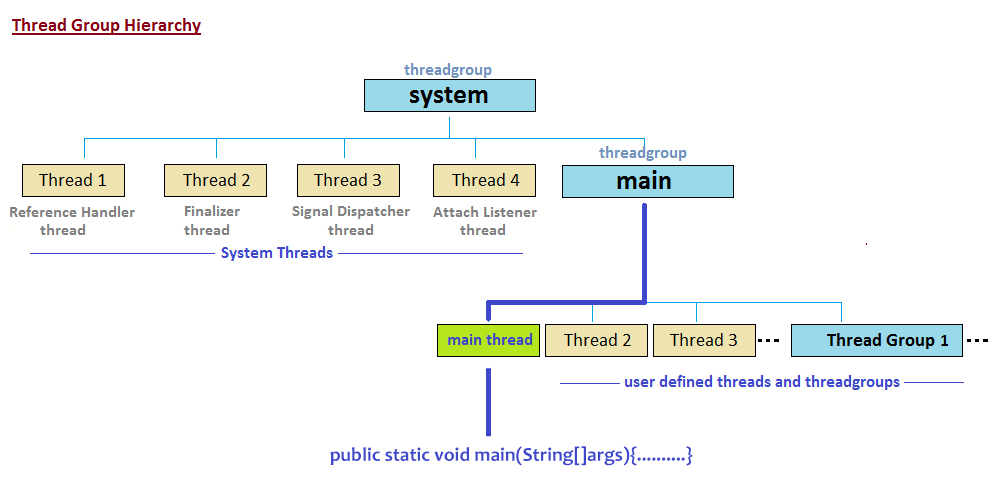Here main() method in itself is a thread because of which you do see Exception in main() while running the program because of which system.main thread exception is thrown sometimes while execution of the program.

### Synchronization

It is the mechanism that bounds the access of multiple threads to share a common resource hence is suggested to be useful where only one thread at a time is granted the access to run over.

It is implemented in the program by using ‘synchronized‘ keyword.

Now let’s finally discuss some advantages and disadvantages of synchronization before implementing the same. For more depth in synchronization, one can also learn object level lock and class level lock and do notice the differences between two to get a fair understanding of the same before implementing the same.

Why synchronization is required?

1. Data inconsistency issues are the primary issue where multiple threads are accessing the common memory which sometimes results in faults in order to avoid that a thread is overlooked by another thread if it fails out.
2. Data integrity
3. To work with a common shared resource which is very essential in the real world such as in banking systems.

Note: Do not go for synchronized keyword unless it is most needed, remember this as there is no priority setup for threads, so if the main thread runs before or after other thread the output of the program would be different.

The biggest advantage of synchronization is the increase in idiotic resistance as one can not choose arbitrarily an object to lock on as a result string literal can not be locked or be the content. Hence, these bad practices are not possible to perform on synchronized method block.

As we have seen humongous advantages and get to know how important it is but there comes disadvantage with it.

Disadvantage: Performance issues will arise as during the execution of one thread all the other threads are put to a blocking state and do note they are not in waiting state. This causes a performance drop if the time taken for one thread is too long.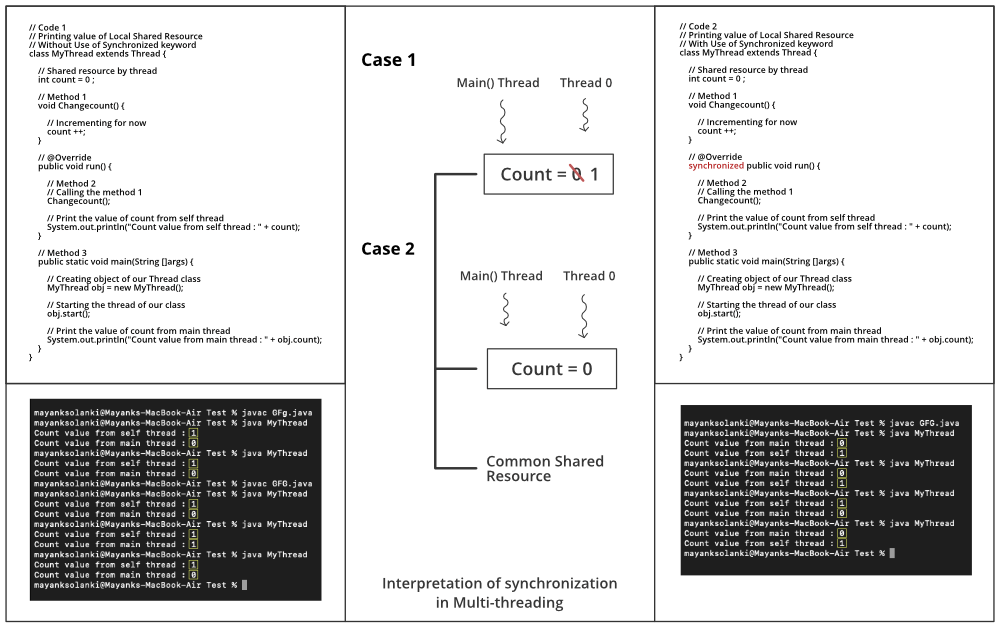As perceived from the image in which we are getting that count variable being shared resource is updating randomly. It is because of multithreading for which this concept becomes a necessity.

• Case 1: If ‘main thread’ executes first then count will be incremented followed by a ‘thread T’ in synchronization
• Case 2: If ‘thread T‘ executes first then count will not increment followed by ‘main thread‘ in synchronization

Implementation: Let us take a sample program to observe this 0 1 count conflict

Example:

## Java

 `// Java Program to illustrate Output Conflict between``// Execution of Main thread vs Thread created` `// count = 1 if main thread executes first``// count = 1 if created thread executes first` `// Importing basic required libraries``import` `java.io.*;``import` `java.util.*;` `// Class 1``// Helper Class extending Thread class``class` `MyThread ``extends` `Thread {` `    ``// Declaring and initializing initial count to zero``    ``int` `count = ``0``;` `    ``// Method 1``    ``// To increment the count above by unity``    ``void` `increment() { count++; }` `    ``// Method 2``    ``// run method for thread invoked after``    ``// created thread has started``    ``public` `void` `run()``    ``{` `        ``// Call method in this method``        ``increment();` `        ``// Print and display the count``        ``System.out.println(``"Count : "` `+ count);``    ``}``}` `// Class 2``public` `class` `GFG {` `    ``// Main driver method``    ``public` `static` `void` `main(String[] args)``    ``{``        ``// Creating the above our Thread class object``        ``// in the main() method``        ``MyThread t1 = ``new` `MyThread();` `        ``// start() method to start execution of created``        ``// thread that will look for run() method``        ``t1.start();``    ``}``}`

Output: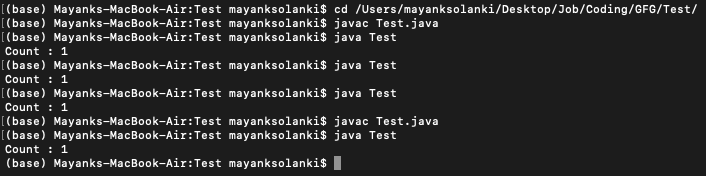Output Explanation:

Here the count is incremented to 1 meaning ‘main thread‘ has executed prior to ‘created thread‘. We have run it many times and compiled and run once again wherein all cases here main thread is executing faster than created thread but do remember output may vary. Our created thread can execute prior to ‘main thread‘ leading to ‘Count : 0’ as an output on the console.

Now another topic that arises in dealing with synchronization in threads is Thread safety in java synchronization is the new concept that arises out in synchronization so let us discuss it considering

• A real-life scenario followed by
• Pictorial representation as an illustration followed by
• Technically description and implementation

Real-life Scenario

Suppose a person is withdrawing some amount of money from the bank and at the same time the ATM card registered with the same account number is carrying on withdrawal operation by some other user. Now suppose if withdrawing some amount of money from net banking makes funds in account lesser than the amount which needed to be withdrawal or the other way. This makes the bank unsafe as more funds are debited from the account than was actually present in the account making the bank very unsafe and is not seen in daily life. So what banks do is that they only let one transaction at a time. Once it is over then another is permitted.

Illustration: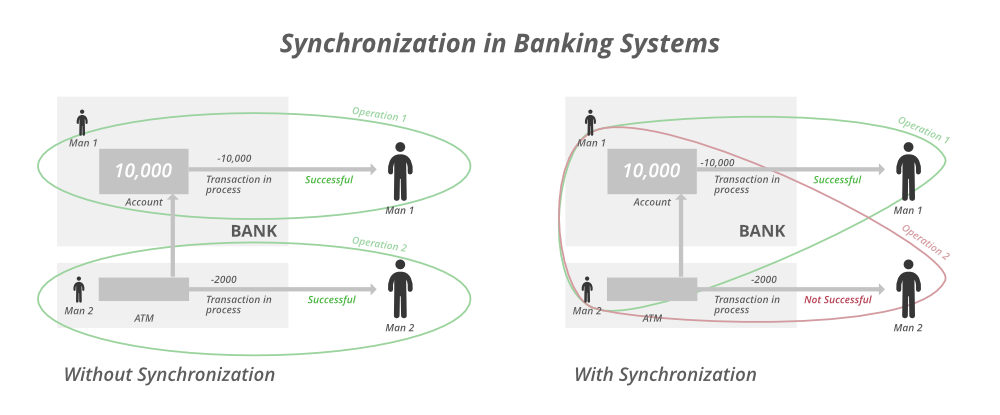Interpreting the same technology as there are two different processes going on which object in case of parallel execution is over headed by threads. Now possessing such traits over threads such that they should look after for before execution or in simpler words making them synchronized. This mechanism is referred to as Thread Safe with the use of the keyword ‘synchronized‘ before the common shared method/function to be performed parallel.

Technical Description:

As we know Java has a feature, Multithreading, which is a process of running multiple threads simultaneously. When multiple threads are working on the same data, and the value of our data is changing, that scenario is not thread-safe, and we will get inconsistent results. When a thread is already working on an object and preventing another thread from working on the same object, this process is called Thread-Safety. Now there are several ways to achieve thread-safety in our program namely as follows:

1. Using Synchronization
2. Using Volatile Keyword
3. Using Atomic Variable
4. Using Final Keyword

Conclusion: Hence, if we are accessing one thread at a time then we can say thread-safe program and if multiple threads are getting accessed then the program is said to be thread-unsafe that is one resource at a time can not be shared by multiple threads at a time.

Implementation:

• Java Program to illustrate Incomplete Thread Iterations returning counter value to Zero irrespective of iteration bound
• Java Program to Illustrate Complete Thread Iterations illustrating join() Method
• Java Program to Illustrate thread-unsafe or non-synchronizing programs as of incomplete iterations
• Java Program to Illustrate Thread Safe And synchronized Programs as of Complete iterations using ‘synchronized‘ Keyword.

Examples

## Java

 `// Example 1``// Java Program to illustrate Incomplete Thread Iterations``// Returning Counter Value to Zero``// irrespective of iteration bound` `// Importing input output classes``import` `java.io.*;` `// Class 1``// Helper Class``class` `TickTock {` `    ``// Member variable of this class``    ``int` `count;` `    ``// Method of this Class``    ``// It increments counter value whenever called``    ``public` `void` `increment()``    ``{``        ``// Increment count by unity``        ``// i.e count = count + 1;``        ``count++;``    ``}``    ``//``}` `// Class 2``// Synchronization demo class``// Main Class``class` `GFG {` `    ``// Main driver method``    ``public` `static` `void` `main(String[] args) ``throws` `Exception``    ``{` `        ``// Creating an object of class TickTock in main()``        ``TickTock tt = ``new` `TickTock();` `        ``// Now, creating an thread object``        ``// using Runnable interface``        ``Thread t1 = ``new` `Thread(``new` `Runnable() {``            ``// Method``            ``// To begin the execution of thread``            ``public` `void` `run()``            ``{` `                ``// Expression``                ``for` `(``int` `i = ``0``; i < ``10000``; i++) {` `                    ``// Calling object of above class``                    ``// in main() method``                    ``tt.increment();``                ``}``            ``}``        ``});` `        ``// Making above thread created to start``        ``// via start() method which automatically``        ``// calls run() method in Ticktock class``        ``t1.start();` `        ``// Print and display the count``        ``System.out.println(``"Count : "` `+ tt.count);``    ``}``}`

## Java

 `// Example 2``// Java Program to Illustrate Complete Thread Iterations``// illustrating join() Method` `// Importing input output classes``import` `java.io.*;` `// Class 1``// Helper Class``class` `TickTock {` `    ``// Member variable of this class``    ``int` `count;` `    ``// Method of this Class``    ``public` `void` `increment()``    ``{` `        ``// Increment count by unity``        ``// whenever this function is called``        ``count++;``    ``}``}` `// Class 2``// Synchronization demo class``// Main Class``class` `GFG {` `    ``// Main driver method``    ``public` `static` `void` `main(String[] args) ``throws` `Exception``    ``{` `        ``// Creating an object of class TickTock in main()``        ``TickTock tt = ``new` `TickTock();` `        ``// Now, creating an thread object``        ``// using Runnable interface``        ``Thread t1 = ``new` `Thread(``new` `Runnable() {``            ``// Method``            ``// To begin the execution of thread``            ``public` `void` `run()``            ``{` `                ``// Expression``                ``for` `(``int` `i = ``0``; i < ``1000``; i++) {` `                    ``// Calling object of above class``                    ``// in main() method``                    ``tt.increment();``                ``}``            ``}``        ``});` `        ``// Making above thread created to start``        ``// via start() method which automatically``        ``// calls run() method``        ``t1.start();` `        ``// Now we are making main() thread to wait so``        ``// that thread t1 completes it job``        ``// using join() method``        ``t1.join();` `        ``// Print and display the count value``        ``System.out.println(``"Count : "` `+ tt.count);``    ``}``}`

## Java

 `// Example 3``// Java Program to Illustrate Thread Unsafe Or``// Non-synchronizing Programs as of Incomplete Iteations``// Without using 'synchronized' program` `// Importing input output classes``import` `java.io.*;` `// Class 1``// Helper Class``class` `TickTock {` `    ``// Member variable of this class``    ``int` `count;` `    ``// Method of this Class``    ``public` `void` `increment()``    ``{` `        ``// Increment count by unity``        ``count++;``    ``}``}` `// Class 2``// Synchronization demo class``// Main Class``class` `GFG {` `    ``// Main driver method``    ``public` `static` `void` `main(String[] args) ``throws` `Exception``    ``{` `        ``// Creating an object of class TickTock in main()``        ``TickTock tt = ``new` `TickTock();` `        ``// Now, creating an thread object``        ``// using Runnable interface``        ``Thread t1 = ``new` `Thread(``new` `Runnable() {``            ``// Method``            ``// To begin the execution of thread``            ``public` `void` `run()``            ``{` `                ``// Expression``                ``for` `(``int` `i = ``0``; i < ``100000``; i++) {` `                    ``// Calling object of above class``                    ``// in main() method``                    ``tt.increment();``                ``}``            ``}``        ``});` `        ``// Now creating another thread and lets check``        ``// how they increment count value running parallelly``        ``// Thread 2``        ``Thread t2 = ``new` `Thread(``new` `Runnable() {``            ``// Method``            ``// To begin the execution of thread``            ``public` `void` `run()``            ``{` `                ``// Expression``                ``for` `(``int` `i = ``0``; i < ``100000``; i++) {` `                    ``// Calling object of above class``                    ``// in main() method``                    ``tt.increment();``                ``}``            ``}``        ``});` `        ``// Making above thread created to start``        ``// via start() method which automatically``        ``// calls run() method``        ``t1.start();``        ``t2.start();` `        ``// Now we are making main() thread to wait so``        ``// that thread t1 completes it job``        ``t1.join();``        ``t2.join();` `        ``// Print and display the count``        ``System.out.println(``"Count : "` `+ tt.count);``    ``}``}`

## Java

 `// Example 4``// Java Program to Illustrate Thread Safe And``// Synchronized Programs as of Complete Iteations``// using 'synchronized' Keyword` `// Importing input output classes``import` `java.io.*;` `// Class 1``// helper Class``class` `TickTock {` `    ``// Member variable of this class``    ``int` `count;` `    ``// Method of this Class``    ``public` `synchronized` `void` `increment()``    ``{` `        ``// Increment count by unity``        ``count++;``    ``}``    ``//``}` `// Class 2``// Synchronization demo class``// Main Class``class` `GFG {` `    ``// Main driver method``    ``public` `static` `void` `main(String[] args) ``throws` `Exception``    ``{` `        ``// Creating an object of class TickTock in main()``        ``TickTock tt = ``new` `TickTock();` `        ``// Now, creating an thread object``        ``// using Runnable interface``        ``Thread t1 = ``new` `Thread(``new` `Runnable() {``            ``// Method``            ``// To begin the execution of thread``            ``public` `void` `run()``            ``{` `                ``// Expression``                ``for` `(``int` `i = ``0``; i < ``100000``; i++) {` `                    ``// Calling object of above class``                    ``// in main() method``                    ``tt.increment();``                ``}``            ``}``        ``});` `        ``// Thread 2``        ``Thread t2 = ``new` `Thread(``new` `Runnable() {``            ``// Method``            ``// To begin the execution of thread``            ``public` `void` `run()``            ``{` `                ``// Expression``                ``for` `(``int` `i = ``0``; i < ``100000``; i++) {` `                    ``// Calling object of above class``                    ``// in main() method``                    ``tt.increment();``                ``}``            ``}``        ``});` `        ``// Making above thread created to start``        ``// via start() method which automatically``        ``// calls run() method``        ``t1.start();``        ``t2.start();` `        ``// Now we are making main() thread to wait so``        ``// that thread t1 completes it job``        ``t1.join();``        ``t2.join();` `        ``// Print and display the count``        ``System.out.println(``"Count : "` `+ tt.count);``    ``}``}`

Output:

Case 1

`Count : 0 `

Case 2

`Count : 10000 `

Case 3

`Count : 151138 `

Case 4

`Count : 200000`

Output Explanation:

In case 1 we can see that count is zero as initialized. Now we have two threads main thread and the thread t1. So there are two threads so now what happens sometimes instance is shared among both of the threads.

In case 1 both are accessing the count variable where we are directly trying to access thread via thread t1.count which will throw out 0 always as we need to call it with the help of object to perform the execution.

Now we have understood the working of synchronization is a thread that is nothing but referred to as a term Concurrency in java which in layman language is executing multiple tasks. Let us depict concurrency in threads with the help of a pictorial illustration.Consider the task of multiplying an array of elements by a multiplier of 2. Now if we start multiplying every element randomly wise it will take a serious amount of time as every time the element will be searched over and computer. By far we have studied multithreading above in which we have concluded to a single line that thread is the backbone of multithreading. So incorporating threads in the above situation as the machine is quad-core we here take 4 threads for every core where we divide the above computing sample set to (1/4)th resulting out in 4x faster computing. If in the above scenario it had taken 4 seconds then now it will take 1 second only. This mechanism of parallel running threads in order to achieve faster and lag-free computations is known as concurrency.

Note: Go for multithreading always for concurrent execution and if not using this concept go for sequential execution despite having bigger chunks of code as safety to our code is the primary issue.

My Personal Notes arrow_drop_up